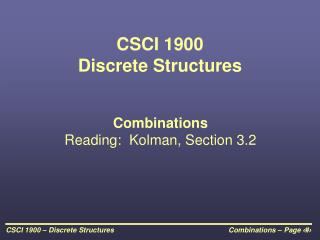DownloadDownload PresentationCSCI 1900 Discrete Structures

# CSCI 1900 Discrete Structures

Télécharger la présentation## CSCI 1900 Discrete Structures

- - - - - - - - - - - - - - - - - - - - - - - - - - - E N D - - - - - - - - - - - - - - - - - - - - - - - - - - -
##### Presentation Transcript

1. CSCI 1900Discrete Structures CombinationsReading: Kolman, Section 3.2

2. Order Doesn’t Matter In the previous section, we looked at two cases where order matters: • Multiplication Principle – duplicates allowed • Permutations – duplicates not allowed

3. Order Doesn’t MatterDuplicates Not Allowed • What if order doesn’t matter, for example, a hand of cards in poker? • Example: the elements 6, 5, and 2 make six possible sequences: 652, 625, 256, 265, 526, and 562 • If order doesn’t matter, these six sequences would be considered the same.

4. Removing Order from Order Notice the example given on the previous slide of the possible sequences involving the elements 6, 5, and 2. The number of arrangements of 6, 5, and 2 equals the number of ways three elements can be ordered, i.e., 3P3. 3P3 = 3!/(3-3)! = 6/1 = 6

5. Removing Order from Order (continued) • Assume that we came up with the number of permutations of three elements from the ten decimal digits 10P3 = 10!/(10-3)! = 10!/7! = 720 • Each subset of three integers from the ten decimal digits would produce 6 sequences. • Therefore, to remove order from the 720 sequences, simply divide by 6 to get 120.

6. Combinations of 3 Digits

7. Combinations • Notation: nCr is called number of combinations of n objects taken r at a time. nCr = n!/[r!  (n – r)!] • Example: How many 5 card hands can be dealt from a deck of 52? 52C5 = 52!/(5!  (52-5)!) • Example: Pick 3 horses from 10 to place in any order • Why are these examples different? • How many ways can a pair of dice come up? • How many dominoes are there in a pack?

8. Order Doesn’t MatterDuplicates Allowed Assume you are walking with your grocery cart past the 2 liter sodas in Walmart. You need to pick up 10 bottles out of: • Coke • Sprite • Dr. Pepper • Pepsi • A&W Root Beer

9. Buying Sodas • You can define how you selected the sodas with a binary string of ones and zeros. • A one indicates you have selected a soda from that category. A zero says that you have moved onto the next category.

10. Moved to Dr. Pepper Last zero is unnecessary Moved to Sprites Moved to Pepsi Moved to A&W 1 1 0 0 1 1 1 0 1 1 0 1 1 1 2 Cokes were picked 3 Dr. Peppers were picked 3 A&W’s were picked No Sprites were picked 2 Pepsis were picked Buying Sodas (continued)

11. Buying Sodas (continued) • This means that a binary pattern of 10 + (5 – 1) = 14 ones and zeros can be used to represent a selection of 10 items from 5 possibilities without worrying about order and allowing duplicates. • This is the same as having 14 elements from which we will select 10 to be set as one, i.e., 14C10 = 14!/(10!  (14 - 10)!) = 1001

12. Order Doesn’t MatterDuplicates Allowed The general formula for order doesn’t matter and duplicates allowed for a selection of r items from a set of n items is: (n + r – 1)Cr